# Mathematics software reviews

Showing 1 to 25 from 114 matches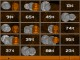Animated Money 1.0 Mathematics Teaches coin recognition and math skills using US and Canadian coins. Shareware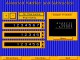Animated Arithmetic 1.0 Mathematics Teaches addition, subtraction, multiplication and division for 1st - 4th grades. Shareware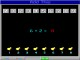Animated Math 1.0 Mathematics Teaches counting, addition and subtraction with animated rewards. Shareware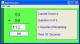Math Flash 4.6 Mathematics Flash card style addition and subtraction practice. Shareware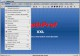MathProf 4.0 Mathematics MathProf is an easy to use mathematics program within approximately 180 subrouti SharewareFree Learn Addition on Orbital One 1.0 Mathematics Learn basic addition in 3D on Orbital One space station. Also includes 27 tests Freeware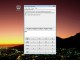Household Calculator 1.0.1.0 Mathematics Household Calculator is a general purpose ergonomic calculator Shareware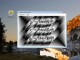Math Center Level 2 1.0.2.1 Mathematics Math software for students studying precalculus and calculus Shareware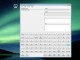Scientific Calculator Decimal 1.0.1.5 Mathematics Scientific Calculator Decimal for scientists, engineers, teachers, and students. Shareware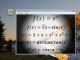Math Center Level 1 1.0.1.9 Mathematics Math software for students studying precalculus Shareware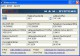ClimeCalc 1.000 Mathematics Pt100 , temperature , relative humidity , dew point , wet-bulb temperature etc. Freeware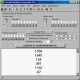Random Number Generator Pro 2.08 Mathematics Generate random numbers. Shareware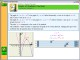MathAid Precalculus 28.63 Mathematics Contain basic concepts, interactive examples, problems and customizable tests DemoMathAid Algebra II 25.63 Mathematics Contain basic concepts, interactive examples, problems and customizable tests DemoMath King 2.00 Mathematics Shareware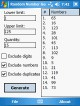Random Number Generator PPC 1.32 Mathematics Generate random numbers Shareware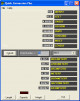Breaktru Quick Conversion 10.4 Mathematics Easily converts several units of length, weight and capacity measures. FreewareNIST-librandomtests 1 Mathematics NIST benchmark for librandomtest.so checking. Includes source and object code. FreewareLeoStatistic 17.4 Mathematics Universal statistical analysis and modeling of experimental and market data. CommercialTimes Tables v2.3 Mathematics Great little program to really help your child learn the Times Tables well Shareware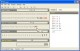BiScope 1.0.0.0 Mathematics Visually explore the relationship between the four main numeral systems. FreewareRapid-Pi 2.1 Mathematics MS Word add-on that provides a faster way of editing mathematical equations Shareware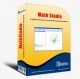Math Studio 3.0 Mathematics Dynamic geometry tool for school math teaching and studying. Demo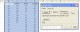Data Pilot 1.03 Mathematics Expand Microsoft Excell data analysis options with Data Pilot FreewareFlashcard Tables 1.11 Mathematics Practice simple mathematical operations for speed. Demo